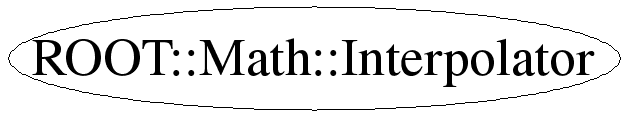# class ROOT::Math::Interpolator

```
Class for performing function interpolation of points.
The class is instantiated with an interpolation methods, passed as an enumeration in the constructor.
See Interpolation::Type for the available interpolation algorithms, which are implemented using GSL.
The class provides additional methods for computing derivatives and integrals of interpolating functions.

This class does not support copying.
@ingroup Interpolation

```

## Function Members (Methods)

public:
 virtual ~Interpolator() double Deriv(double x) const double Deriv2(double x) const double Eval(double x) const double Integ(double a, double b) const ROOT::Math::Interpolator Interpolator(unsigned int ndata = 0, ROOT::Math::Interpolation::Type type = Interpolation::CSPLINE) ROOT::Math::Interpolator Interpolator(const vector& x, const vector& y, ROOT::Math::Interpolation::Type type = Interpolation::CSPLINE) bool SetData(const vector& x, const vector& y) bool SetData(unsigned int ndata, const double* x, const double* y) string Type() const string TypeGet() const
private:
 ROOT::Math::Interpolator Interpolator(const ROOT::Math::Interpolator&) ROOT::Math::Interpolator& operator=(const ROOT::Math::Interpolator&)

## Data Members

private:
 ROOT::Math::GSLInterpolator* fInterp pointer to GSL interpolator class

## Class Charts## Function documentation

Interpolator(unsigned int ndata = 0, ROOT::Math::Interpolation::Type type = Interpolation::CSPLINE)
```Constructs an interpolator class from  number of data points and with Interpolation::Type type.
The data can be set later on with the SetData method.
In case the data size is not known, better using the default of zero or the next constructor later on.
The defult interpolation type is Cubic spline

```
Interpolator(const vector<double>& x, const vector<double>& y, ROOT::Math::Interpolation::Type type = Interpolation::CSPLINE)
```Constructs an interpolator class from vector of data points \f\$ (x_i, y_i )\f\$ and with Interpolation::Type type.
The method will compute a continous interpolating function \f\$ y(x) \f\$ such that \f\$ y_i = y ( x_i )\f\$.
The defult interpolation type is Cubic spline

```
virtual ~Interpolator()
Interpolator(const ROOT::Math::Interpolator& )
``` usually copying is non trivial, so we make this unaccessible
```
bool SetData(const vector<double>& x, const vector<double>& y)
```Set the data vector ( x[] and y[] )
To be efficient, the size of the data must be the same of the value used in the constructor (ndata)
If this is not  the case a new re-initialization is performed with the new data size

```
bool SetData(unsigned int ndata, const double* x, const double* y)
```Set the data vector ( x[] and y[] )
To be efficient, the size of the data must be the same of the value used when  constructing the class (ndata)
If this is not  the case a new re-initialization is performed with the new data size.

```
double Eval(double x) const
```Return the interpolated value at point x

```
double Deriv(double x) const
```Return the derivative of the interpolated function at point x

```
double Deriv2(double x) const
```Return the second derivative of the interpolated function at point x

```
double Integ(double a, double b) const
```Return the Integral of the interpolated function over the range [a,b]

```
std::string Type()
```Return the type of interpolation method

```
std::string TypeGet()

Last update: root/mathmore:\$Id: Interpolator.h 21637 2008-01-11 09:56:31Z moneta \$
Copyright (c) 2004 ROOT Foundation, CERN/PH-SFT *

This page has been automatically generated. If you have any comments or suggestions about the page layout send a mail to ROOT support, or contact the developers with any questions or problems regarding ROOT.